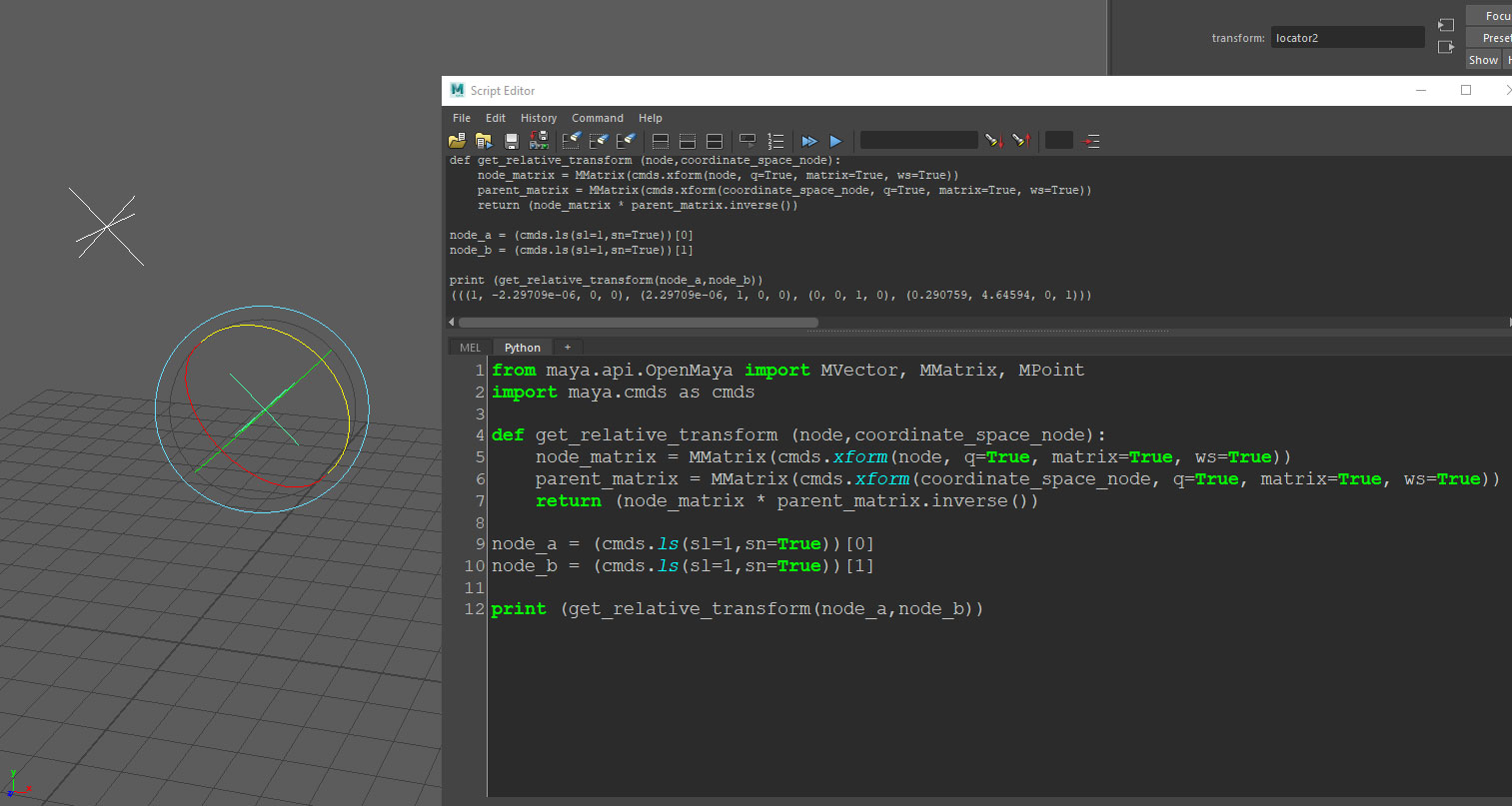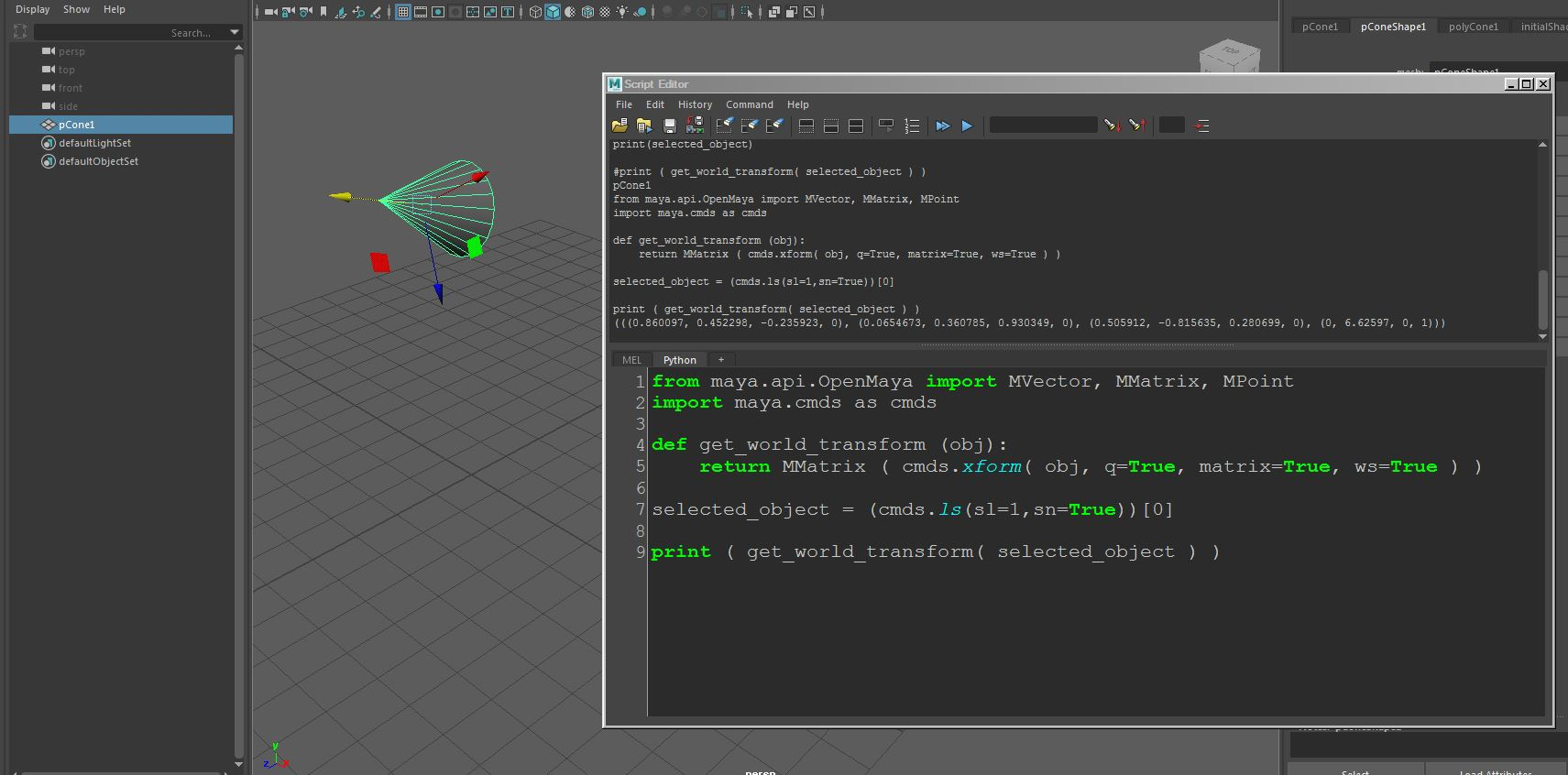## A collection of Python snippets for 3D

If you’re interested in taking the first step into Python for 3D software,
Or simply would like to browse some script examples, your welcome to visit my Gist page,
It contains a useful library of Python code example for Blender, Maya, 3ds max and Unreal engine:
https://gist.github.com/CGLion

## Maya Python scripting – Iterate through the timeline frames

Software:
Maya 2018

The following Python script iterates though Maya’s timeline frames, and for each frame creates a new cube, and aligns it’s position to the selected animated locator.

* There is probably a nicer way to set one object’s position according to anothers but haven’t found it yet (not finding enough examples of the cmds.xform command…) so sorry for that..

```import maya.cmds as cmds

selection = cmds.ls(sl=1,sn=True)
for frame in range(1,80):
cmds.currentTime(frame)
newCube = cmds.ls (cmds.polyCube( sx=1, sy=1, sz=1), long=True)
posX = cmds.getAttr(selection+'.translateX')
posY = cmds.getAttr(selection+'.translateY')
posZ = cmds.getAttr(selection+'.translateZ')
cmds.setAttr(newCube+'.translateX',posX)
cmds.setAttr(newCube+'.translateY',posY)
cmds.setAttr(newCube+'.translateZ',posZ)```## Maya Python scripting – Getting an object’s transform matrix relative to another object’s coordinates

Software:
Maya 2018

How to get an object transformation matrix relative to another object’s coordinates:
* The following script requires selecting 2 objects, the function will return the transform matrix of the first object relative to the transform matrix of the second.

```from maya.api.OpenMaya import MVector, MMatrix, MPoint
import maya.cmds as cmds

def get_relative_transform (node,coordinate_space_node):
node_matrix = MMatrix(cmds.xform(node, q=True, matrix=True, ws=True))
parent_matrix = MMatrix(cmds.xform(coordinate_space_node, q=True, matrix=True, ws=True))
return (node_matrix * parent_matrix.inverse())

node_a = (cmds.ls(sl=1,sn=True))
node_b = (cmds.ls(sl=1,sn=True))

print (get_relative_transform(node_a,node_b))```## Maya Python scripting – Getting an Object’s world-space transform matrix

Software:
Maya 2018

How to get an object transformation matrix in world space coordinates:

```from maya.api.OpenMaya import MVector, MMatrix, MPoint
import maya.cmds as cmds

def get_world_transform (obj):
return MMatrix ( cmds.xform( obj, q=True, matrix=True, ws=True ) )

selected_object = (cmds.ls(sl=1,sn=True))

print ( get_world_transform( selected_object ) )```